﻿ 一种数字有源相控阵天线的测量方法 A New Method for Measuring Digital Active Phased Array Antenna

Journal of Antennas
Vol. 07  No. 03 ( 2018 ), Article ID: 28280 , 8 pages
10.12677/JA.2018.73003

A New Method for Measuring Digital Active Phased Array Antenna

Jian Ding, Shuji Hao, Libin Lv

National Key Laboratory of Electromagnetic Environment, China Research Institute of Radiowave Propagation, Qingdao Shandong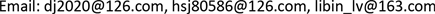Received: Dec. 1st, 2018; accepted: Dec. 21st, 2018; published: Dec. 28th, 2018ABSTRACT

A new method is proposed to solve the measurement problem of digital active phased array antenna by using the modified coding algorithm. Fixing the probe in the mid-field of the phased array antenna, using the controllable excitation amplitude and phase of the digital active phased array antenna, the excitation amplitude and phase are coded, and the initial excitation is quickly recovered. The radiation characteristics of the measured antenna are obtained by using the active element pattern and the initial excitation. The simulation results of two digital active phased array antenna models verify the correctness and efficiency of the proposed method.

Keywords:Digital Active Phased Array Antenna, Active Element Pattern, Mid-Field, Orthogonal CodingCopyright © 2018 by authors and Hans Publishers Inc.1. 引言

2. 测量方法模型

$E\left(\theta ,\varphi \right)={\sum }_{q=1}^{N}{V}_{q}{g}_{u}^{q}\left(\theta ,\varphi \right){\text{e}}^{jk\stackrel{^}{r}{r}_{q}}$ (1)

$E\left(\theta ,\varphi \right)={g}_{av}^{i}\left(\theta ,\varphi \right){\sum }_{n=1}^{{N}_{i}}{V}_{n}{\text{e}}^{jk\stackrel{^}{r}{r}_{n}}+{\sum }_{m=1}^{{N}_{e}}{V}_{m}{g}_{u}^{m}\left(\theta ,\varphi \right){\text{e}}^{jk\stackrel{^}{r}{r}_{m}}$ (3)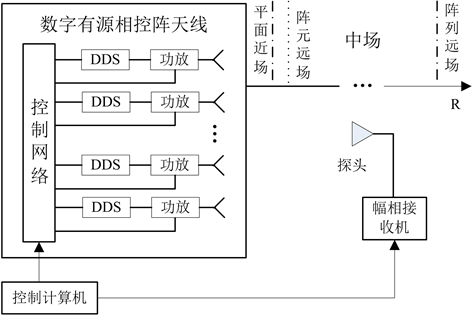Figure 1. Measurement model of digital active phased array antenna

$y={\sum }_{q=1}^{N}{V}_{q}{c}_{q}$ (2)

${c}_{q}=\frac{{\text{e}}^{-jk{d}_{q}}}{{d}_{q}}{g}_{u}^{q}\left({\stackrel{^}{d}}_{q}\right)\cdot {f}_{p}\left(-{\stackrel{^}{d}}_{q}\right)$ (3)

3. 变型的编码算法的测量原理

${H}_{{2}^{k}}=\left[\begin{array}{cc}{H}_{{2}^{k-1}}& {H}_{{2}^{k-1}}\\ {H}_{{2}^{k-1}}& -{H}_{{2}^{k-1}}\end{array}\right]$ (4)

${a}_{q}=\left\{\begin{array}{l}t\left(q\right){V}_{q},\text{\hspace{0.17em}}\text{\hspace{0.17em}}\text{\hspace{0.17em}}\text{\hspace{0.17em}}H\left(m,n+1\right)=+1\\ {V}_{q},\text{\hspace{0.17em}}\text{\hspace{0.17em}}\text{\hspace{0.17em}}\text{\hspace{0.17em}}\text{\hspace{0.17em}}\text{\hspace{0.17em}}\text{\hspace{0.17em}}\text{\hspace{0.17em}}\text{\hspace{0.17em}}\text{\hspace{0.17em}}H\left(m,n+1\right)=-1\end{array}$ (5)

$y\left(m\right)={\sum }_{q=1}^{N}{a}_{q}{c}_{q}={\sum }_{q=1}^{N}\left(\frac{1+H\left(m,n+1\right)}{2}{V}_{q}+\frac{1-H\left(m,n+1\right)}{2}t\left(q\right){w}_{q}\right){c}_{q}$ (6)

${H}_{M}^{-1}=\frac{1}{M}{H}_{M}$ (7)

$z\left(q\right)=\frac{1}{M}{\sum }_{m=1}^{N}H\left(m,n+1\right)y\left(m\right),\text{\hspace{0.17em}}\text{\hspace{0.17em}}\text{\hspace{0.17em}}q=1,2,\cdots ,N$ (8)

$z\left(q\right)=\frac{\left(1-t\left(q\right)\right){V}_{q}{c}_{q}}{2},\text{\hspace{0.17em}}\text{\hspace{0.17em}}\text{\hspace{0.17em}}q=1,2,\cdots ,N$ (9)

${V}_{q}=\frac{2\ast z\left(q\right)}{\left(1-t\left(q\right)\right){c}_{q}},\text{\hspace{0.17em}}\text{\hspace{0.17em}}\text{\hspace{0.17em}}q=1,2,\cdots ,N$ (10)

4. 仿真结果分析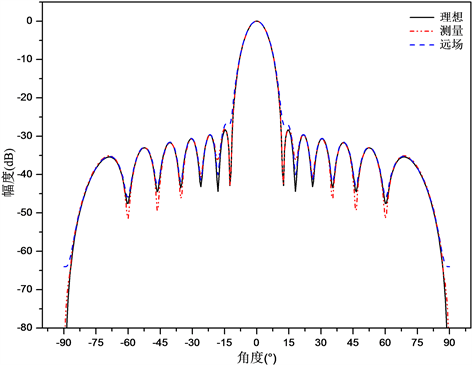Figure 2. Radiation pattern of 14-element linear array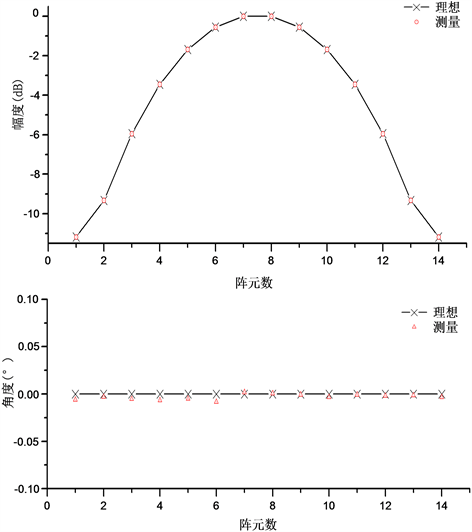Figure 3. Amplitude and phase distribution of elements in 14-element linear array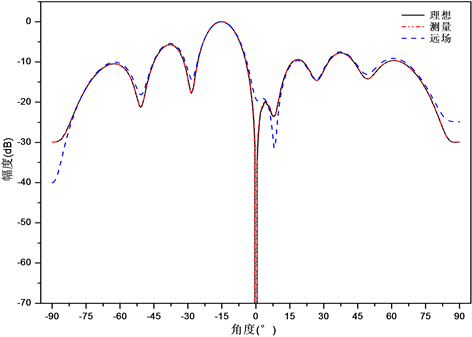Figure 4. Scanning radiation pattern of 7 × 7 planar array

5. 结论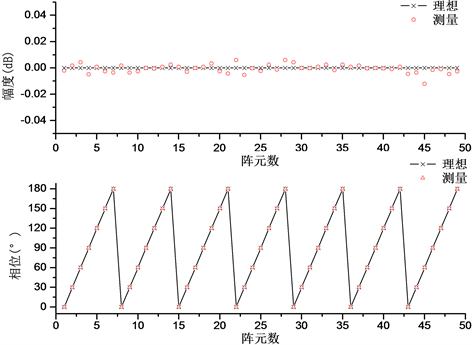Figure 5. Amplitude and phase distribution of elements in 7 × 7 planar array

A New Method for Measuring Digital Active Phased Array Antenna[J]. 天线学报, 2018, 07(03): 17-24. https://doi.org/10.12677/JA.2018.73003

1. 1. Evans, G.E. (1990) Antenna Measurement Techniques. Artech House, Inc., London.

2. 2. Isernia, T., Leone, G., et al. (1996) Radiation Pattern Evaluation from Near-Field Intensities on Planes. IEEE Transactions on Antennas and Prop-agation, 44, 701-710. https://doi.org/10.1109/8.496257

3. 3. Patton, W.T. and Yorinks, L.H. (1999) Near-Field Alignment of Phased Array Antenna. IEEE Transactions on Antennas and Propagation, 47, 584-591. https://doi.org/10.1109/8.768795

4. 4. 张俭, 熊继衮. 相控阵天线的聚焦法[J]. 系统工程与电子技术, 1990(1): 36-44.

5. 5. 高火涛, 吴世才, 侯杰昌, 等. 高频地波雷达天线阵方向图的近场聚焦技术[J]. 武汉大学学报(理学版), 2001, 47(5): 545-549.

6. 6. Takahashi, T., Miyashita, H. and Konishi, Y. (2006) Theoretical Study on Measurement Accuracy of Rotating Element Electric Field Vector (REV) Method. Electronics and Communications in Japan, Part 1, 90, 22-33. https://doi.org/10.1002/ecja.20239

7. 7. Takahashi, T., Konishi, Y. and Makino, S. (2008) Fast Measurement Technique for Phased Array Calibration. IEEE Transactions on Antennas and Propagation, 56, 1888-1899. https://doi.org/10.1109/TAP.2008.924682

8. 8. Ovidio, M.B. and Migliore, M.D. (2005) Accurate Diagnosis of Conformal Arrays from Near-Field Data Using the Matrix Method. IEEE Transactions on Antennas and Propagation, 53, 1114-1120. https://doi.org/10.1109/TAP.2004.842656

9. 9. Shang, J.-P., Deng, Y.-B., Jiang, S., et al. (2008) Study on a Fast Measurement Method of Phased Array Antennas. The 8th International Symposium on Antennas, Propagation, and EM Theory, Kunming, 2-5 November 2008, 161-165.

10. 10. 尚军平, 傅德民, 等. 基于最佳配相控制的相控阵天线快速测量方法研究[J]. 电波科学学报, 2008, 23(2): 331-334.

11. 11. 尚军平, 傅德民, 徐平. 基于控制电路编码算法的相控阵天线快速测量[J]. 电波科学学报, 2010, 25(1): 73-76.

12. 12. Lier, E., Zemlyansky, M., et al. (2010) Phased Array Calibration and Characterization Based on Orthogonal Coding: Theory and Experimental Validation. 2010 IEEE In-ternational Symposium on Phased Array Systems and Technology, Waltham, 12-15 October 2010, 271-278. https://doi.org/10.1109/ARRAY.2010.5613357

13. 13. David, F.K. (1993) Array Antenna Pattern Methods That In-clude Mutual Coupling Effects. IEEE Transactions on Antennas and Propagation, 41, 1625-1632. https://doi.org/10.1109/8.273305

14. 14. Pozar, D.M. (1994) The Active Element Pattern. IEEE Transactions on Antennas and Propagation, 42, 1176-1178. https://doi.org/10.1109/8.310010